# Transient Dynamic Response of a Clamp Beam with Different InertiaThis test lets you check analysis results for a beam with different inertia, in the context of a transient dynamic response case. You will use a 1D mesh.
This test proposed by AFNOR is used to validate the transient dynamic response solve algorithms.Reference:

Guide de validation des prologiciels de calcul de structures, AFNOR Technique, SFM pp188-189.

##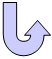Specifications

### Geometry Specifications

 Beams AC and DB: l = AC = DB = 1.25 m area = 2.872e-3 m2 Iy = 1.943e-5 m4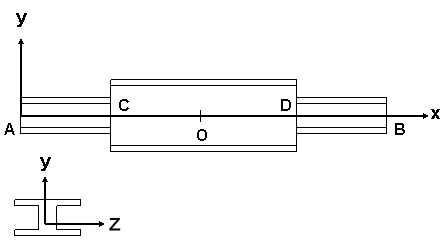Beams CD: L = CD = 2.5 m area = 5.356e-3 m2 Iy = 8.356e-5 m4 The structure and the load being symmetrical, only one half of the beam is modeled.

### Analysis Specifications

 Young Modulus (material): E = 200 GPa Masses for Frequency Case: Edge AC: Line mass density = 22.402 kg/m Edge OC: Line mass density = 42.398 kg/m Mesh Specifications: Beam mesh with a mesh size equal to 62.5 mm Restraints: Clamp on A Edges AC and CO: Rx = 0 Point O: Tx = Ty = Rx = Ry = Rz = 0 Loads: q1 = 10000 N_m all beam long t1 = 2.38e-2 s t2 = 5.95e-2 s Global damping = 0 %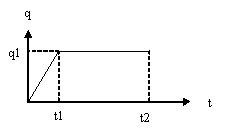##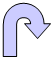Results

### Frequency Response

 Bending mode Frequency [Hz] Error [%] Analytical solution Computed results 1 63.009 62.8796 0.21

### Transient Dynamic Response

 Time [s] Point localization [mm] Displacement V [mm] Error [%] Analytical Solution Computed results 0.0595 2437.5 2.469 2.467 0.09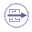To Perform the Test:

The Transient_dynamic_response_beam_inertia.CATAnalysis document presents the complete analysis of the transient dynamic response case.

To compute the case, proceed as follow:

1. Open the CATAnalysis document.

2. Compute the Frequency case, and generate an image called Deformed mesh.